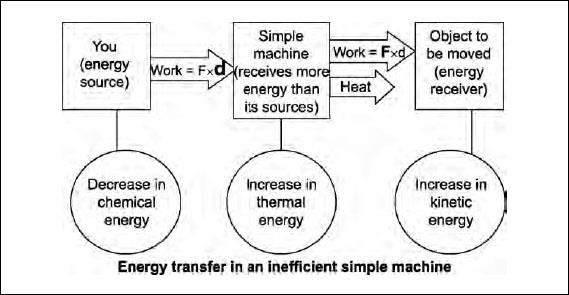NextPrevious

# How can a machine with a mechanical advantage less than or equal to one be useful?

If a simple machine has a mechanical advantage less than one then you exert a larger force on it than it puts on whatever object it contacts. The output force is less than the input force. How can that be useful? It’s because the distance moved (output distance) is larger than what you move (input distance). And, therefore, the output speed is also greater. A baseball bat, tennis racket, and golf club can be considered simple machines in which high speed of the end of the implement is desired.

Are machines with mechanical advantage equal to one useful? They are because they change the direction the force is exerted, which often makes it easier for the person to exert that force.Close

This is a web preview of the "The Handy Physics Answer Book" app. Many features only work on your mobile device. If you like what you see, we hope you will consider buying. Get the App